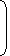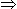# Tricks to solve Problems on TrainsProblems on trains are very common in competitive exams. Various types of questions are asked on trains. Questions on trains are solved using the concept of time, speed and distance i.e. we use the formulas of time, speed and distance to solve questions on trains.

• Speed=Distance/Time
• If Time is constant,SpeedDistance
• If Distance is constant,Speed1/Time
• If Speed is constant,DistanceTime
• km/hr to m/s conversion:
 a km/hr =a x 5m/s. 18
• m/s to km/hr conversion:
 a m/s =a x 18km/hr. 5
• Time taken by a train of length l meters to pass a pole or standing man or a signal post is equal to the time taken by the train to cover l meters.
• Time taken by a train of length l meters to pass a stationery object of length b meters is the time taken by the train to cover (l + b) meters.
• Suppose two trains or two objects bodies are moving in the same direction at u m/s and v m/s, where u > v, then their relative speed is = (u – v) m/s.
• Suppose two trains or two objects bodies are moving in opposite directions at u m/s and v m/s, then their relative speed is = (u + v) m/s.
• If two trains of length a meters and b meters are moving in opposite directions at u m/s and v m/s, then:
 The time taken by the trains to cross each other = (a + b) sec. (u + v)
• If two trains of length a meters and b meters are moving in the same direction at u m/s and v m/s, then:
 The time taken by the faster train to cross the slower train = (a + b) sec. (u – v)

If two trains (or bodies) start at the same time from points A and B towards each other and after crossing they take a and b sec in reaching B and A respectively, then:

(A’s speed) : (B’s speed) = ( b :  a)

### Problems on Trains – General Examples

1) A train moving at speed of 80 km/hr crosses a pole in 7 seconds. Find the length of the train.

1. 150 m
2. 165 m
3. 175 m
4. 170 m

The correct option is (C).

Length of the train is equal to the distance covered by train to cross the pole. So, we will find the distance travelled by the train in 7 seconds by applying the following formula:

Distance= Speed x Time

Speed is given in Km/hr so we will convert it into m/s as answers are given in meters.

Speed=90*= 25 m/s

Time = 7 seconds

Distance = 25 * 7= 175 meters

2) A train of length 200 meters crosses a man running at 10 km/hr in the same direction in 10 seconds. What is the speed of the train?

1. 72 km/hr
2. 95 km/hr
3. 85 km/hr
4. 82 km/hr

The correct option is (D).

When the train and man are moving in same direction then relative speed will be the difference between their individual speeds. In this problem the other way to find the relative speed is to divide the distance covered (length of train) by the time taken by the train to cross the man.

Relative Speed=We will convert it into Km/hr= 72 km/hr

Now, let the speed of the train is X km/hr. So, the relative speed, 72 km/hr = X km/hr ? 10 km/hr

X-10=72

X= 72+10

X= 82 km/hr

3)

A train passes a station platform in 36 seconds and a man standing on the platform in 20 seconds. If the speed of the train is 54 km/hr, what is the length of the platform?
 A. 120 m B. 240 m C. 300 m D. None of these

The correct Option is B

 Speed =54 x 5m/sec = 15 m/sec. 18

Length of the train = (15 x 20)m = 300 m.

Let the length of the platform be x metres.

 Then, x + 300 = 15 36x + 300 = 540x = 240 m.

Correct! Wrong!

Correct! Wrong!

Correct! Wrong!

Correct! Wrong!

#### Two trains of equal lengths take 10 seconds and 15 seconds respectively to cross a telegraph post. If the length of each train be 120 metres, in what time (in seconds) will they cross each other travelling in opposite direction?

Correct! Wrong!

speed,time ,distance QUIZ
great job